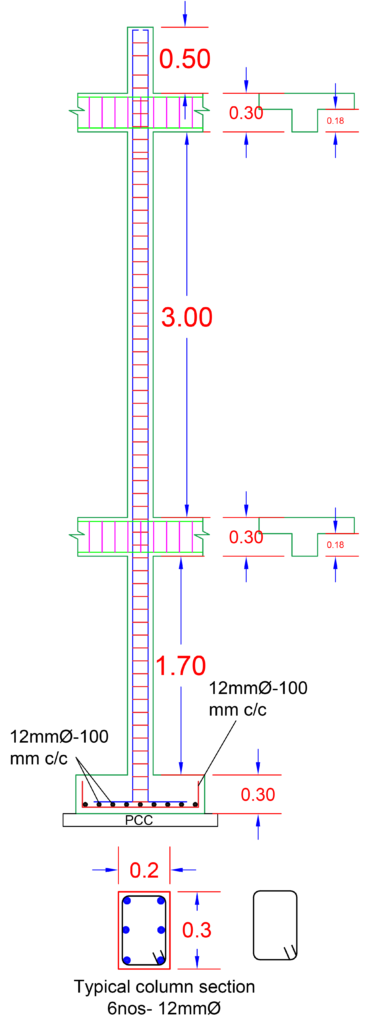# Calculation of Column Cutting length and Weight Calculation for Column Main Bar ( Column BBS )

Given Value From Drawing

• Footing Depth      – 300mm• Cover                        – Footing 50mm, Column -40mm
• Beam Depth           – 300 mm
• UG Column             – 1700 mm
• Floor To Floor Height – 3000 mm
• Parapet Wall                  – 500 mm

Now Calculate Cutting Length Of Column

Cutting Length = (Footing Depth-Cover – M Bar – D Bar) + L Length + UG + No of Beam + No of Floor + Parapet + Developed Length + Lapping Length – Band
*M Bar = Footing Main bar, D Bar = Distribution Bar of Footing

Cutting Length = (300-50-12-12) + 300 + 1700 + (2 x 300) + (1 x 3000) + 500 + 50 – (2x2x12)
Cutting Length = (300-74) + 2000 + 600 + 3000 + 550 – 48

Cutting Length = 226 + 6150 – 48

Cutting Length = 6376- 48

Cutting Length of 1 Bar = 6328 mm = 6.33 m.

Now Calculate weight of One Bar

One bar Length is 6.33 m

As we know formula for weight calculation of steel bar for 1 meter is = D2/162

Now we using 12 mm dia of bar for Column.

Than weight for 1 meter bar of 12 mm dia is

1 meter (12mm) = 122/162

1 meter (12mm) = 144/162

1 meter (12mm) = 0.888 KG/ m

Now our Cutting length of column for 1 Bar is 6.33 m

One bar weight  = 6.33 *0.888  = 5.62Kg

Now as we in our column design 6 Nos of Bar in single column

as we Calculated 1 Bar weight is 5.62 kg

Than 6 Nos of Bar is 5.62 x 6 = 33.82 kg per column

Stirrups Calculation for Column & Beam Click Below Option

Rectangular Stirrups Cutting Length Calculation  With Excel Sheet

Circular Stirrups Cutting Length Calculation  With Excel Sheet

#### Column BBS Calculation Excel Sheet####Visit ourChannel for more information.

Tutorials tips# Amit Sah

### 9 Responses

1.Elaf khan says:

Thank you tutorials tips civil for giving me this easy tips how to calculate Such a things thanks

•Anurag says:

sir is BBS mein aapne joggle length be consider kia hai kya ?

•Tutorial Tips Civil says:

No, But Aap 1D le sakte hai

2.fahad khan says:

what does it mean for 2*2*12??

•malik wajid says:

There are two 90 degree bends. For 90, we take 2d and multiply by the total bends. 2*2d

3.Vicky Kumar Das says:

thanks sir

4.Dipesh Rathore says:

Thanks sir

5.Hukum chand says:

Thanks sir

•Tutorial Tips Civil says:

thanks to you for your comment.